# Exact sequence

(diff) ← Older revision | Latest revision (diff) | Newer revision → (diff)

A sequence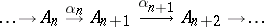of objects of an Abelian categoryand of morphismssuch that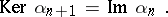An exact sequence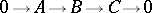is called short, and consists of an object, a subobjectof it and the corresponding quotient object.

Exact sequences often occur and are often used in (co)homological considerations. There are, e.g., the long homology exact sequence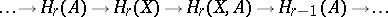of a pair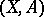,a subspace of, and the long cohomology exact sequence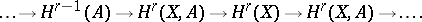Analogous long exact sequences occur in a variety of other homology and cohomology theories. Cf. Homology theory; Cohomology; Cohomology sequence; Homology sequence, and various articles on the (co)homology of various kinds of objects, such as Cohomology of algebras; Cohomology of groups; Cohomology of Lie algebras.

An exact sequence of the formis sometimes called a left short exact sequence and one of the form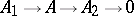a right short exact sequence. The exact sequence of a morphism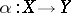in an Abelian category is the exact sequenceHow to Cite This Entry:
Exact sequence. Encyclopedia of Mathematics. URL: http://encyclopediaofmath.org/index.php?title=Exact_sequence&oldid=46866
This article was adapted from an original article by V.E. Govorov (originator), which appeared in Encyclopedia of Mathematics - ISBN 1402006098. See original article